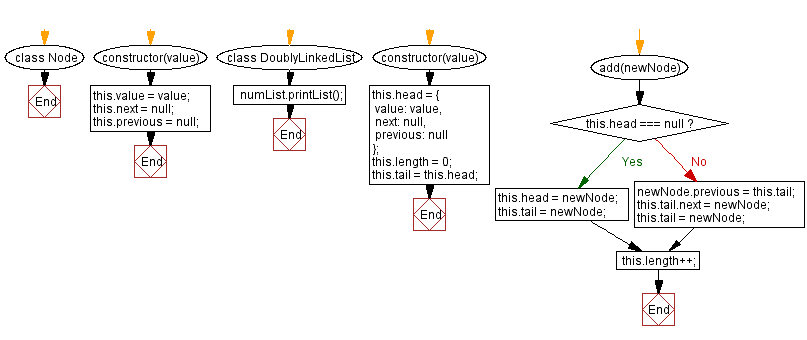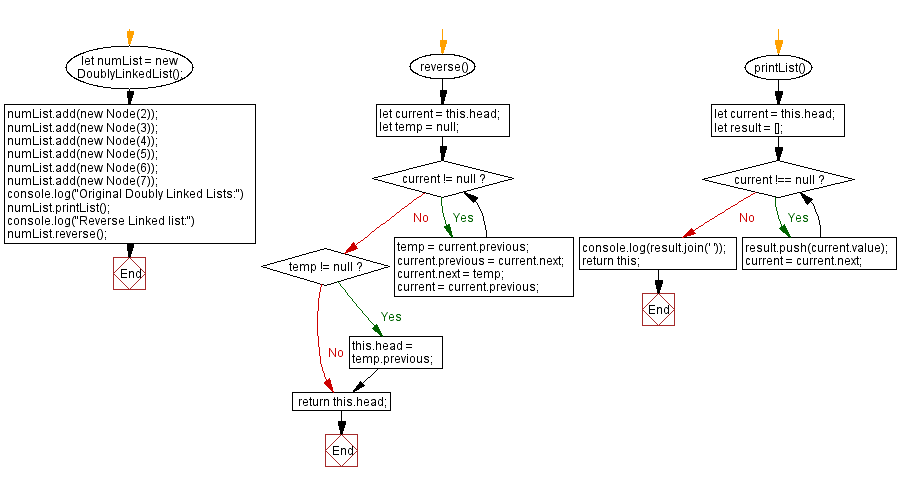# JavaScript Exercises: Print a Doubly Linked lists in reverse order

## JavaScript Data Structures: Exercise-9 with Solution

Write a JavaScript program to create a Doubly Linked lists of n nodes and display it in reverse order.

Sample Solution:

JavaScript Code:

``````class Node {
constructor(value) {
this.value = value;
this.next = null;
this.previous = null;
}
}

constructor(value) {
value: value,
next: null,
previous: null
};
this.length = 0;
}

this.tail = newNode;
}
else
{
newNode.previous = this.tail;
this.tail.next = newNode;
this.tail = newNode;
}

this.length++;
}

reverse() {
let temp = null;
while (current != null) {
temp = current.previous;
current.previous = current.next;
current.next = temp;
current = current.previous;
}
if (temp != null) {
}
}

printList() {
let result = [];
while (current !== null) {
result.push(current.value);
current = current.next;
}
console.log(result.join(' '));
return this;
}
}

numList.printList();
numList.reverse();
numList.printList();
```
```

Sample Output:

```Original Doubly Linked Lists:
2 3 4 5 6 7
7 6 5 4 3 2
```

Flowchart:Live Demo:

See the Pen javascript-doubly-linked-list-exercise-9 by w3resource (@w3resource) on CodePen.

Improve this sample solution and post your code through Disqus

Linked List Previous: Get the value of a node at a given position in a Doubly Linked List.

What is the difficulty level of this exercise?

Test your Programming skills with w3resource's quiz.

﻿

## JavaScript: Tips of the Day

Creates an array of elements, ungrouping the elements in an array produced by zip and applying the provided function

Example:

```const tips_unzip = (arr, fn) =>
arr
.reduce(
(acc, val) => (val.forEach((v, i) => acc[i].push(v)), acc),
Array.from({
length: Math.max(...arr.map(x => x.length))
}).map(x => [])
)
.map(val => fn(...val));

console.log(tips_unzip([[2, 15, 200], [3, 25, 300]], (...args) => args.reduce((acc, v) => acc + v, 0)));
```

Output:

```[5, 40, 500]
```